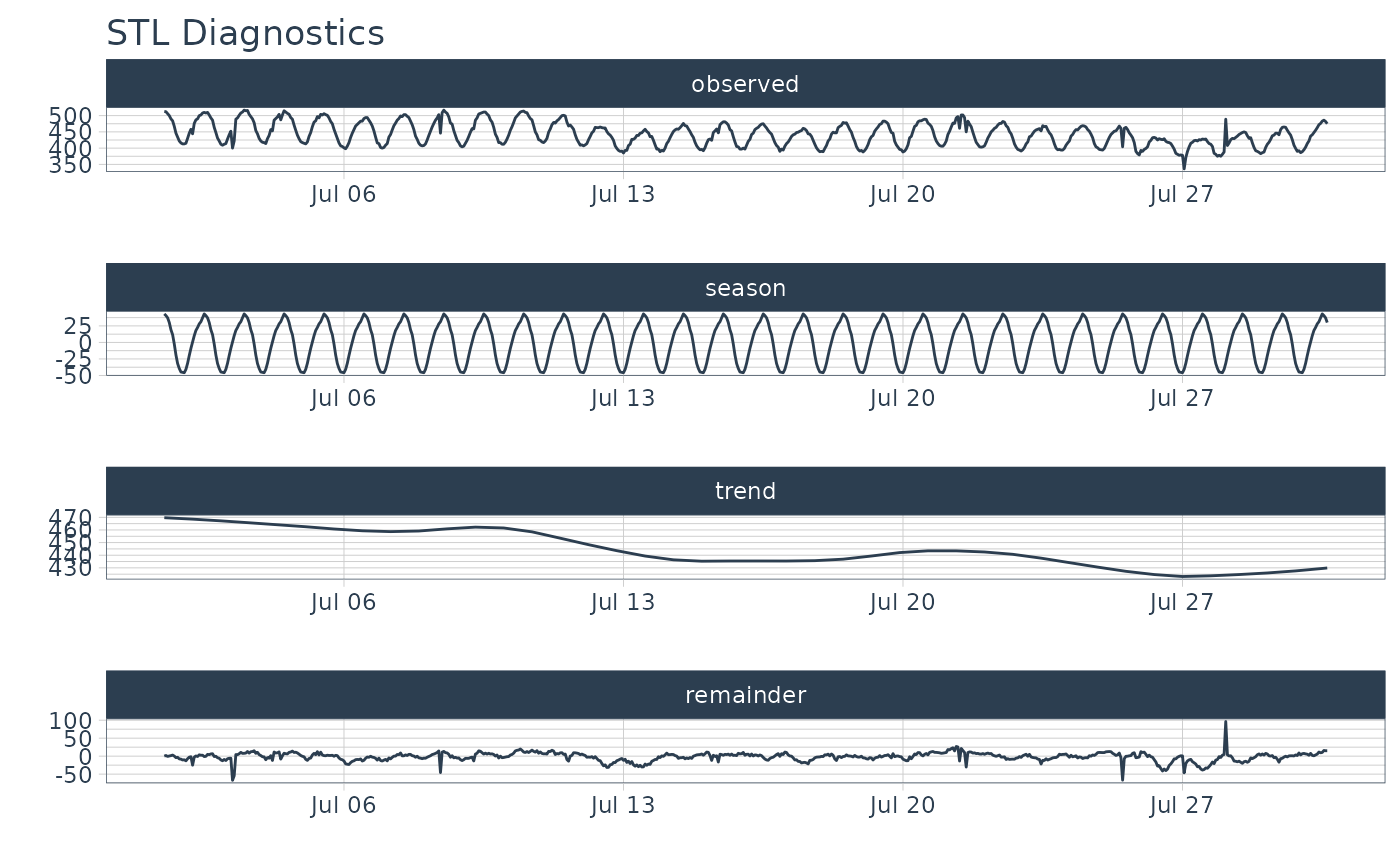An interactive and scalable function for visualizing time series STL Decomposition. Plots are available in interactive plotly (default) and static ggplot2 format.

## Usage

plot_stl_diagnostics(
.data,
.date_var,
.value,
.facet_vars = NULL,
.feature_set = c("observed", "season", "trend", "remainder", "seasadj"),
.frequency = "auto",
.trend = "auto",
.message = TRUE,
.facet_scales = "free",
.line_color = "#2c3e50",
.line_size = 0.5,
.line_type = 1,
.line_alpha = 1,
.title = "STL Diagnostics",
.x_lab = "",
.y_lab = "",
.interactive = TRUE
)

## Arguments

.data

A tibble or data.frame with a time-based column

.date_var

A column containing either date or date-time values

.value

A column containing numeric values

.facet_vars

One or more grouping columns that broken out into ggplot2 facets. These can be selected using tidyselect() helpers (e.g contains()).

.feature_set

The STL decompositions to visualize. Select one or more of "observed", "season", "trend", "remainder", "seasadj".

.frequency

Controls the seasonal adjustment (removal of seasonality). Input can be either "auto", a time-based definition (e.g. "2 weeks"), or a numeric number of observations per frequency (e.g. 10). Refer to tk_get_frequency().

.trend

Controls the trend component. For STL, trend controls the sensitivity of the lowess smoother, which is used to remove the remainder.

.message

A boolean. If TRUE, will output information related to automatic frequency and trend selection (if applicable).

.facet_scales

Control facet x & y-axis ranges. Options include "fixed", "free", "free_y", "free_x"

.line_color

Line color.

.line_size

Line size.

.line_type

Line type.

.line_alpha

Line alpha (opacity). Range: (0, 1).

.title

Plot title.

.x_lab

Plot x-axis label

.y_lab

Plot y-axis label

.interactive

If TRUE, returns a plotly interactive plot. If FALSE, returns a static ggplot2 plot.

## Value

A plotly or ggplot2 visualization

## Details

The plot_stl_diagnostics() function generates a Seasonal-Trend-Loess decomposition. The function is "tidy" in the sense that it works on data frames and is designed to work with dplyr groups.

STL method:

The STL method implements time series decomposition using the underlying stats::stl(). The decomposition separates the "season" and "trend" components from the "observed" values leaving the "remainder".

Frequency & Trend Selection

The user can control two parameters: .frequency and .trend.

1. The .frequency parameter adjusts the "season" component that is removed from the "observed" values.

2. The .trend parameter adjusts the trend window (t.window parameter from stl()) that is used.

The user may supply both .frequency and .trend as time-based durations (e.g. "6 weeks") or numeric values (e.g. 180) or "auto", which automatically selects the frequency and/or trend based on the scale of the time series.

## Examples

library(dplyr)
library(timetk)

# ---- SINGLE TIME SERIES DECOMPOSITION ----
m4_hourly %>%
filter(id == "H10") %>%
plot_stl_diagnostics(
date, value,
# Set features to return, desired frequency and trend
.feature_set = c("observed", "season", "trend", "remainder"),
.frequency   = "24 hours",
.trend       = "1 week",
.interactive = FALSE)
#> frequency = 24 observations per 24 hours
#> trend = 168 observations per 1 week# ---- GROUPS ----
m4_hourly %>%
group_by(id) %>%
plot_stl_diagnostics(
date, value,
.feature_set = c("observed", "season", "trend"),
.interactive = FALSE)
#> frequency = 24 observations per 1 day
#> trend = 336 observations per 14 days
#> frequency = 24 observations per 1 day
#> trend = 336 observations per 14 days
#> frequency = 24 observations per 1 day
#> trend = 336 observations per 14 days
#> frequency = 24 observations per 1 day
#> trend = 336 observations per 14 days